SLVSBB7E May   2012  – November 2015

PRODUCTION DATA.

1. Features
2. Applications
3. Description
4. Revision History
5. Pin Configuration and Functions
6. Specifications
7. Detailed Description
1. 7.1 Overview
2. 7.2 Functional Block Diagram
3. 7.3 Feature Description
4. 7.4 Device Functional Modes
8. Application and Implementation
1. 8.1 Application Information
2. 8.2 Typical Applications
1. 8.2.1 CCM Application
2. 8.2.2 DCM Application
9. Power Supply Recommendations
10. 10Layout
11. 11Device and Documentation Support
12. 12Mechanical, Packaging, and Orderable Information

• DRB|8
• DRB|8

## 8 Application and Implementation

NOTE

Information in the following applications sections is not part of the TI component specification, and TI does not warrant its accuracy or completeness. TI’s customers are responsible for determining suitability of components for their purposes. Customers should validate and test their design implementation to confirm system functionality.

### 8.1 Application Information

The TPS54061 is a 60-V, 200-mA step-down regulator with an integrated high-side and low-side MOSFET. This device is typically used to convert a higher DC voltage to a lower DC voltage with a maximum available output current of 200 mA. Example applications are: Low-Power Standby or Bias Voltage Supplies, 4-20 mA Current Loop Powered Sensors, Industrial Process Control, Metering, and Security Systems or an efficient high voltage linear regulator replacement. Use the following design procedure to select component values for the TPS54061. This procedure illustrates the design of a high-frequency switching regulator. These calculations can be done with the aid of the excel spreadsheet tool SLVC431. Alternatively, use the WEBENCH software to generate a complete design. The WEBENCH software uses an iterative design procedure and accesses a comprehensive database of components when generating a design.

### 8.2 Typical Applications

#### 8.2.1 CCM Application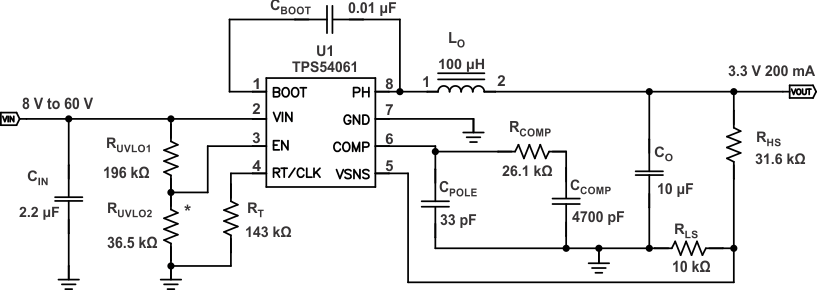Figure 20. CCM Application Schematic

#### 8.2.1.1 Design Requirements

This example details the design of a continuous conduction mode (CCM) switching regulator design using ceramic output capacitors. If a low-output current design is needed, see DCM Application. A few parameters must be known in order to start the design process. These parameters are typically determined at the system level. For this example, we will start with the following known parameters:

### Table 1. Design Parameters

PARAMETERS VALUES
Output Voltage 5.0 V
Transient Response 50 to 150mA load step ΔVOUT = 4%
Maximum Output Current 200 mA
Input Voltage 24 V nom. 8 V to 60 V
Output Voltage Ripple 0.5% of VOUT
Start Input Voltage (rising VIN) 7.50 V
Stop Input Voltage (falling VIN) 6.50 V

#### 8.2.1.2.1 Selecting the Switching Frequency

The first step is to decide on a switching frequency for the regulator. Typically, the user will want to choose the highest switching frequency possible because this will produce the smallest solution size. The high switching frequency allows for lower valued inductors and smaller output capacitors compared to a power supply that switches at a lower frequency. The switching frequency is limited by the minimum on-time of the internal power switch, the maximum input voltage, the output voltage and the frequency shift limitation.

Equation 6 and Equation 7 must be used to find the maximum switching frequency for the regulator, choose the lower value of the two results. Switching frequencies higher than these values will result in pulse skipping or a lack of overcurrent protection during short circuit conditions. The typical minimum on-time, tonmin, is 120 ns for the TPS54061. To ensure overcurrent runaway does not occur during short circuits in your design, use Equation 7 to determine the maximum switching frequency. With a maximum input voltage of 60 V, inductor resistance of 0.77 Ω, high-side switch resistance of 3.0 Ω, low-side switch resistance of 1.5 Ω, a current limit value of 350 mA and a short circuit output voltage of 0.1 V, the maximum switching frequency is 524 kHz and 1003 kHz in each case respectively. A switching frequency of 400 kHz is used. To determine the timing resistance for a given switching frequency, use Equation 5. The switching frequency is set by resistor RT shown in Figure 20. RT is calculated to be 142 kΩ. A standard value of 143 kΩ is used.

#### 8.2.1.2.2 Output Inductor Selection (LO)

To calculate the minimum value of the output inductor, use Equation 8. KIND is a coefficient that represents the amount of inductor ripple current relative to the maximum output current. The inductor ripple current will be filtered by the output capacitor. Therefore, choosing high inductor ripple currents will impact the selection of the output capacitor because the output capacitor must have a ripple current rating equal to or greater than the inductor ripple current. In general, the inductor ripple value is at the discretion of the designer; however, the following guidelines may be used. Typically it is recommended to use KIND values in the range of 0.2 to 0.4; however, for designs using low-ESR output capacitors such as ceramics and low output currents, a KIND value as high as 1 may be used. In a wide input voltage regulator, it is best to choose an inductor ripple current on the larger side. This allows the inductor to still have a measurable ripple current with the input voltage at its minimum. For this design example, use KIND of 0.4 and the minimum inductor value is calculated to be 97 µH. For this design, a standard 100 µH value was chosen. It is important that the RMS current and saturation current ratings of the inductor not be exceeded. The RMS and peak inductor current can be found from Equation 10 and Equation 11.

For this design, the RMS inductor current is 200 mA and the peak inductor current is 239 mA. The chosen inductor is a Würth 74408943101. It has a saturation current rating of 680 mA and an RMS current rating of 520 mA. As the equation set demonstrates, lower ripple currents will reduce the output voltage ripple of the regulator but will require a larger value of inductance. Selecting higher ripple currents will increase the output voltage ripple of the regulator but allow for a lower inductance value. The current flowing through the inductor is the inductor ripple current plus the average output current. During power-up, faults or transient load conditions, the inductor current can increase above the peak inductor current level calculated above. In transient conditions, the inductor current can increase up to the switch current limit of the device. For this reason, the most conservative approach is to specify an inductor with a saturation current rating equal to or greater than the switch current limit rather than the calculated peak inductor current.

Equation 8.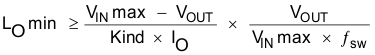Equation 9.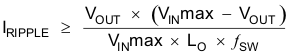Equation 10.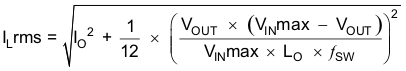Equation 11.#### 8.2.1.2.3 Output Capacitor

There are three primary considerations for selecting the value of the output capacitor. The output capacitor will determine the modulator pole, the output voltage ripple, and how the regulator responds to a large change in load current. The output capacitance needs to be selected based on the most stringent of these three criteria. The desired response to a large change in the load current is the first criteria. The output capacitor needs to supply the load with current until the regulator increases the inductor current. This situation would occur if there are desired hold-up times for the regulator where the output capacitor must hold the output voltage above a certain level for a specified amount of time after the input power is removed. The regulator also will temporarily not be able to supply sufficient output current if there is a large, fast increase in the current needs of the load such as transitioning from no load to a full load. The regulator usually needs two or more clock cycles for the control loop to see the change in load current and output voltage and adjust the duty cycle to react to the change. The output capacitor must be sized to supply the extra current to the load until the control loop responds to the load change. The output capacitance must be large enough to supply the difference in current for 2 clock cycles while only allowing a tolerable amount of droop in the output voltage. Equation 15 shows the minimum output capacitance necessary to accomplish this, where ΔIout is the change in output current, ƒsw is the regulators switching frequency and ΔVout is the allowable change in the output voltage.

For this example, the transient load response is specified as a 4% change in Vout for a load step from 50 mA to 150 mA. For this example, ΔIOUT = 0.150 –0.05 = 0.10 and ΔVOUT = 0.04 × 3.3 = 0.132.

Using these values gives a minimum capacitance of 3.79 µF. This does not take the ESR of the output capacitor into account in the output voltage change. For ceramic capacitors, the ESR is usually small enough to ignore in this calculation. Aluminum electrolytic and tantalum capacitors have higher ESR that should be taken into account.

The low side FET of the regulator emulates a diode so it can not sink current so any stored energy in the inductor will produce an output voltage overshoot when the load current rapidly decreases, as in Figure 28. The output capacitor must also be sized to absorb energy stored in the inductor when transitioning from a high load current to a lower load current. The excess energy that gets stored in the output capacitor will increase the voltage on the capacitor. The capacitor must be sized to maintain the desired output voltage during these transient periods. Equation 14 is used to calculate the minimum capacitance input the output voltage overshoot to a desired value, where LO is the value of the inductor, IOH is the output current under heavy load, IOL is the output under light load, VO+ΔVO is the final peak output voltage, and Vi is the initial capacitor voltage. For this example, the worst case load step will be from 150 mA to 50 mA. The output voltage will increase during this load transition and must be limited to 4% of the output voltage to satisy the design goal. This will make VO+ΔVO = 1.04 × 3.3 = 3.432 V. VO is the initial capacitor voltage which is the nominal output voltage of 3.3 V. Using these numbers in Equation 14 yields a minimum capacitance of 2.25 µF.

Equation 13 calculates the minimum output capacitance needed to meet the output voltage ripple specification, where fSW is the switching frequency, Vripple is the maximum allowable output voltage ripple, and Iripple is the inductor ripple current. Equation 13 yields 1.48 µF. Equation 16 calculates the maximum ESR an output capacitor can have to meet the output voltage ripple specification. Equation 16 indicates the ESR should be less than 0.160 Ω.

The most stringent criteria for the output capacitor is 3.79 µF of capacitance to maintain the output voltage regulation during an load transient.

Additional capacitance de-ratings for aging, temperature and DC bias will increase this minimum value. For this example, a 10-µF, 10-V X5R ceramic capacitor with 0.003 Ω of ESR in a 1206 package is used.

Capacitors generally have limits to the amount of ripple current they can handle without failing or producing excess heat. An output capacitor that can support the inductor ripple current must be specified. Some capacitor data sheets specify the Root Mean Square (RMS) value of the maximum ripple current.

Equation 12 can be used to calculate the RMS ripple current the output capacitor needs to support. For this example, Equation 12 yields 10.23 mA.

Equation 12.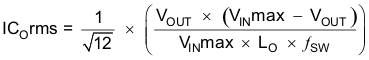Equation 13.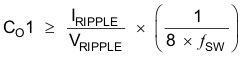Equation 14.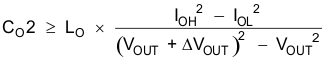Equation 15.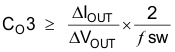Equation 16.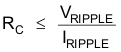#### 8.2.1.2.4 Input Capacitor

The TPS54061 requires a high-quality ceramic, type X5R or X7R, input decoupling capacitor of at least 1 µF of effective capacitance. The effective capacitance includes any deration for DC bias effects. The voltage rating of the input capacitor must be greater than the maximum input voltage. The capacitor must also have an RMS current rating greater than the maximum RMS input current. The input RMS current can be calculated using Equation 17. The value of a ceramic capacitor varies significantly over temperature and the dc bias applied to the capacitor. The capacitance variations with temperature can be minimized by selecting a dielectric material that is stable over temperature. X5R and X7R ceramic dielectrics are usually selected for power regulator capacitors because they have a high capacitance to volume ratio and are fairly stable over temperature. The effective value of a capacitor decreases as the DC bias across a capacitor increases. For this example design, a ceramic capacitor with at least a 60-V voltage rating is required to support the maximum input voltage. The input capacitance value determines the input ripple voltage of the regulator. The input voltage ripple can be calculated by rearranging Equation 18.

Using the design example values, Ioutmax = 200 mA, CIN = 2.2 µF, ƒSW = 400 kHz, yields an input voltage ripple of 56.8 mV and an RMS input ripple current of 98.5 mA.

Equation 17.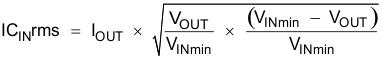Equation 18.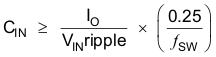#### 8.2.1.2.5 Bootstrap Capacitor Selection

A 0.01-µF ceramic capacitor must be connected between the BOOT and PH pins for proper operation. TI recommends using a ceramic capacitor with X5R or better grade dielectric. The capacitor should have 10 V or higher voltage rating.

#### 8.2.1.2.6 UVLO Set Point

The UVLO can be adjusted using an external voltage divider on the EN pin of the TPS54061. The UVLO has two thresholds, one for power-up when the input voltage is rising and one for power-down or brown outs when the input voltage is falling. For the example design, the supply should turn on and start switching once the input voltage increases above 7.50 V (enabled). After the regulator starts switching, it should continue to do so until the input voltage falls below 6.50 V (UVLO stop). The programmable UVLO and enable voltages are set by connecting resistor divider between Vin and ground to the EN pin. Equation 2 and Equation 3 can be used to calculate the resistance values necessary. For example, a 196-kΩ resistor between Vin and EN and a 36.5-kΩ resistor between EN and ground are required to produce the 7.50 and 6.50 volt start and stop voltages. See the Enable and Adjusting Undervoltage Lockout section for additional considerations in high input voltage applications.

#### 8.2.1.2.7 Output Voltage and Feedback Resistors Selection

For the example design, 10 kΩ was selected for RLS. Using Equation 1, RHS is calculated as 31.46 kΩ. The nearest standard 1% resistor is 31.6 kΩ.

#### 8.2.1.2.8 Closing the Loop

There are several methods used to compensate DC-DC regulators. The method presented here is easy to calculate and ignores the effects of the slope compensation that is internal to the device. Because the slope compensation is ignored, the actual crossover frequency will usually be lower than the crossover frequency used in the calculations. This method assume the crossover frequency is between the modulator pole and the ESR zero and the ESR zero is at least 10 times greater the modulator pole.

To get started, the modulator pole, fpole, and the ESR zero, fzero must be calculated using Equation 19 and Equation 20. For Cout, use a derated value of 6.0 µF. Use Equation 21 and Equation 22, to estimate a starting point for the crossover frequency, fco, to design the compensation. For the example design, fpole is 1015 Hz and fzero is 5584 kHz.

Equation 21 is the geometric mean of the modulator pole and the ESR zero and Equation 22 is the mean of modulator pole and the switching frequency. Equation 21 yields 119.2 kHz and Equation 22 gives 17.9 kHz. Use a frequency near the lower value of Equation 21 or Equation 22 for an initial crossover frequency.

For this example, fco of 17.9 kHz is used. Next, the compensation components are calculated. A resistor in series with a capacitor is used to create a compensating zero. A capacitor in parallel to these two components forms the compensating pole.

To determine the compensation resistor, RCOMP, use Equation 23. Assume the power stage transconductance, gmps, is 1.00 A/V. The output voltage, Vo, reference voltage, VREF, and amplifier transconductance, gmea, are 3.3 V, 0.8 V and 108 µA/V, respectively.

RCOMP is calculated to be 25.9 kΩ, use the nearest standard value of 26.1 kΩ. Use Equation 24 to set the compensation zero equal to the modulator pole frequency. Equation 24 yields a 3790 pF for capacitor CCOMP and a 4700 pF is chosen. Use the larger value of Equation 25 and Equation 26 to calculate the CPOLE value, to set the compensation pole. Equation 26 yields 30.5 pF so the nearest standard of 33 pF is selected.

Equation 19.Equation 20.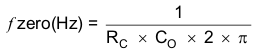Equation 21.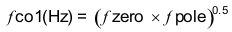Equation 22.Equation 23.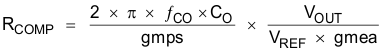Equation 24.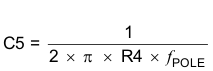Equation 25.Equation 26.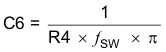#### 8.2.1.3 Application CurvesFigure 21. Efficiency vs Output Current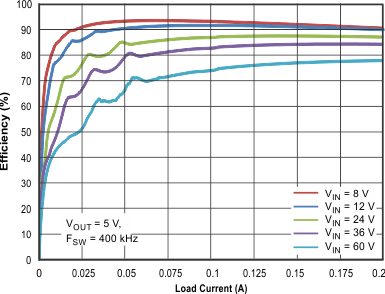Figure 23. Efficiency vs Output Current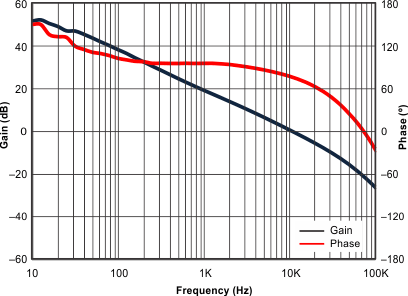Figure 25. Gain vs Phase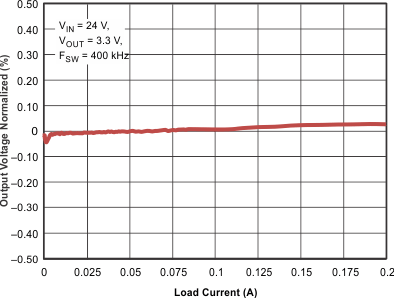Figure 27. Output Voltage vs Output Current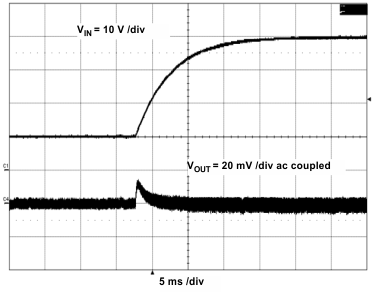Figure 29. Line Transient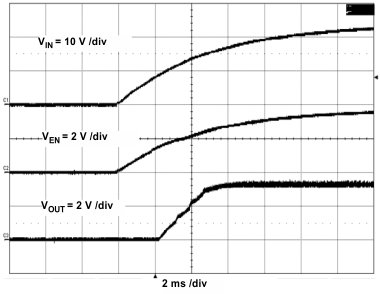Figure 31. Start-Up With VINFigure 33. Input Ripple in CCM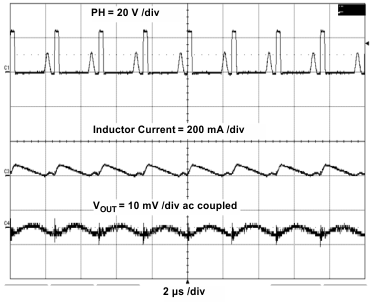Figure 35. Output Ripple in DCMFigure 37. Output Ripple Skip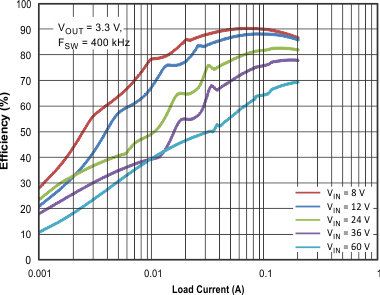Figure 22. Efficiency vs Output Current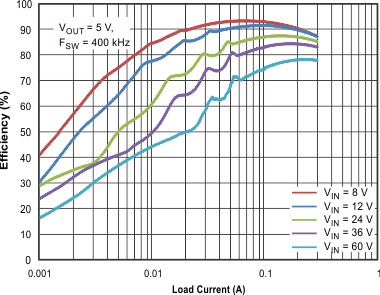Figure 24. Efficiency vs Output Current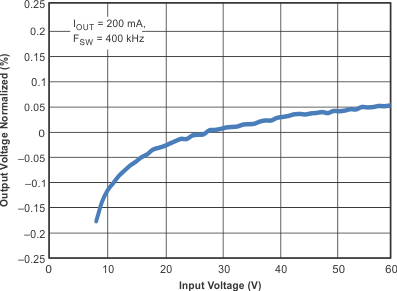Figure 26. Output Voltage vs Input Voltage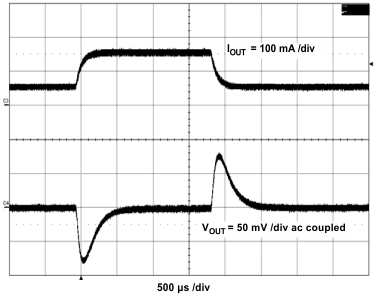Figure 28. Load TransientFigure 30. Start-Up With ENA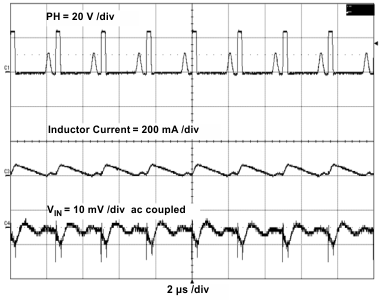Figure 32. Input Ripple in DCM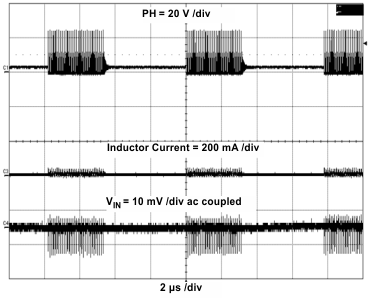Figure 34. Input Ripple Skip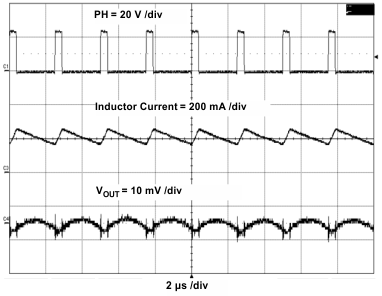Figure 36. Output Ripple in CCM

#### 8.2.2 DCM Application

It is most desirable to have a power supply that is efficient and has a fixed switching frequency at low output currents. A fixed frequency power supply will have a predictable output voltage ripple and noise. Using a traditional continuous conduction mode (CCM) design method to calculate the output inductor will yield a large inductance for a low output current supply. Using a CCM inductor will result in a large sized supply or will affect efficiency from the large dc resistance an alternative is to operate in discontinuous conduction mode (DCM). Use the procedure below to calculate the components values for designing a power supply operating in discontinuous conduction mode. The advantage of operating a power supply in DCM for low output current is the fixed switching frequency, lower output inductance, and lower DC resistance on the inductor. Use the frequency shift and skip equations to estimate the maximum switching frequency.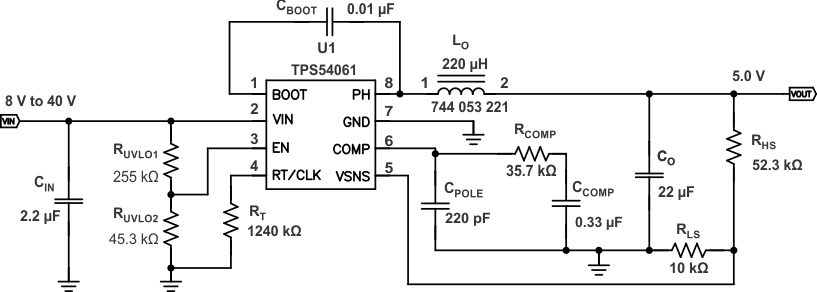Figure 38. DCM Application Schematic

#### 8.2.2.1 Design Requirements

This example details the design of a low-output current, fixed-switching regulator design using ceramic output capacitors. A few parameters must be known in order to start the design process. These parameters are typically determined at the system level. For this example, we will start with the following known parameters:

### Table 2. Design Parameters

DESIGN PARAMETERS VALUES
Output Voltage 5.0 V
Transient Response 37.5 to 75-mA load step ΔVOUT = 4%
Maximum Output Current 75 mA
Minimum Output Currert 1 mA
Input Voltage 24 V nom. 8 V to 40 V
Output Voltage Ripple 1 % of VOUT
Switching Frequency 50 kHz
Start Input Voltage (rising VIN) 8 V
Stop Input Voltage (falling VIN) 6.8 V

#### 8.2.2.2.1 Designing an Efficient, Low-Output Current Power Supply at a Fixed Switching Frequency

The TPS54061 is designed for applications which require a fixed operating frequency and low output voltage ripple at low-output currents, thus, the TPS54061 does not have a pulse skip mode at light loads. Because the device has a minimum controllable on time, there is an output current at which the power supply will pulse skip. To ensure that the supply does not pulse skip at output current of the application the inductor value will be need to be selected greater than a minimum value. The minimum inductance needed to maintain a fixed switching frequency at the minimum load is calculated to be 227 µH using Equation 27. Because the equation is ideal and was derived without losses, assume the minimum controllable light load on time, tonminll, is 180 ns. To maintain DCM operation the inductor value and output current need to stay below a maximum value. The maximum inductance is calculated to be 250 µH using Equation 28. A 744053221 inductor from Würth Elektronik is selected. If CCM operation is necessary, use the previous design procedure.

Use Equation 29, to make sure the minimum current limit on the high-side power switch is not exceeded at the maximum output current. The peak current is calculated as 244 mA and is lower than the 350 mA current limit. To determine the RMS current for the inductor and output capacitor, it is necessary to calculate the duty cycle. The duty cycle, D1, for a step down regulator in DCM is calculated in Equation 30. D1 is the portion of the switching cycle the high side power switch is on, and is calculated to be 0.1345. D2 is the portion of the switching cycle the low-side power switch is on, and is calculated to be 0.5111.

Using the Equation 32 and Equation 33, the RMS current of the inductor and output capacitor are calculated, to be 0.1078 A and 0.0774 A respectively. Select components that ratings exceed the calculated RMS values. Calculate the output capacitance using the Equation 34 to Equation 36 and use the largest value, Vripple is the steady-state voltage ripple and deltaV is voltage change during a transient. A minimum of 7.5-µF capacitance is calculated. Additional capacitance de-ratings for aging, temperature and DC bias should be factored in which increases this minimum value. For this example, a 22-µF 10-V X7R ceramic capacitor with 5-mΩ ESR is used. To have a low-output ripple power supply use a low ESR capacitor. Use Equation 37 to estimate the maximum ESR for the output capacitor. Equation 38 and Equation 39 estimate the RMS current and capacitance for the input capacitor. An RMS current of 38.7 mA and capacitance of 1.56 µF is calculated. A 2.2-µF 100-V/X7R ceramic is used for this example.

Equation 27.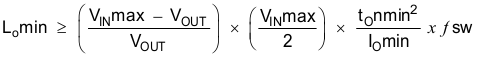Equation 28.Equation 29.Equation 30.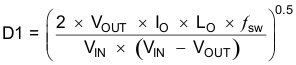Equation 31.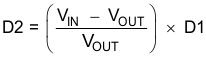Equation 32.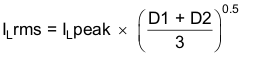Equation 33.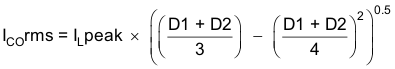Equation 34.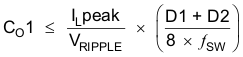Equation 35.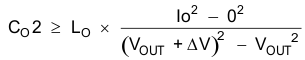Equation 36.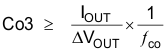Equation 37.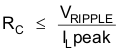Equation 38.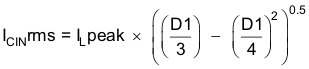Equation 39.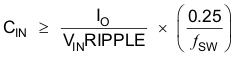#### 8.2.2.2.2 Closing the Feedback Loop

The method presented here is easy to calculate and includes the effect of the slope compensation that is internal to the TPS54061. This method assumes the crossover frequency is between the modulator pole and the ESR zero and the ESR zero is at least 10 times greater than the modulator pole. Once the output components are determined, use the equations below to close the feedback loop. A current mode controlled power supply operating in DCM has a transfer function which includes an ESR zero and pole as shown in Equation 40. To calculate the current mode power stage gain, first calculate, Kdcm, the DCM gain, and Fm, the modulator gain, using Equation 41 and Equation 42. Kdcm and Fm are 32.4 and 0.475 respectively. The location of the pole and ESR zero are calculated using Equation 43 and Equation 44 . The pole and zero are 491 Hz and 2.8 MHz, respectively. Use the lower value of Equation 45 and Equation 46 as a starting point for the crossover frequency. Equation 45 is the geometric mean of the power stage pole and the ESR zero and Equation 46 is the mean of power stage pole and the switching frequency. The crossover frequency is chosen as 5 kHz from Equation 46.

To determine the compensation resistor, RCOMP, use Equation 47. Assume the power stage transconductance, gmps, is 1.0 A/V. The output voltage, VO, reference voltage, VREF, and amplifier transconductance, gmea, are
5.0 V, 0.8 V and 108 µA/V, respectively. RCOMP is calculated to be 38.3 kΩ; use the nearest standard value of 35.7 kΩ. Use Equation 48 to set the compensation zero to equalthe modulator pole frequency. Equation 48 yields 290 nF for compensating capacitor CCOMP, and a 330 nF is used. Use the larger value of Equation 49 or Equation 50 to calculate the CPOLE, which sets the compensation pole. Equation 50 yields 178 pF standard value of 220 pF is selected.

Equation 40.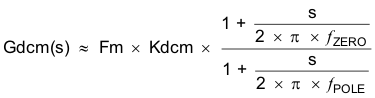Equation 41.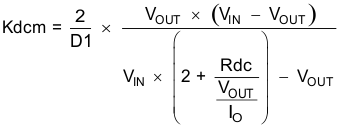Equation 42.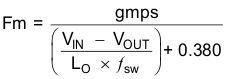Equation 43.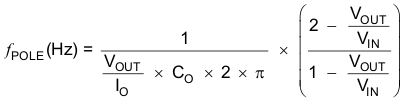Equation 44.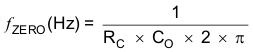Equation 45.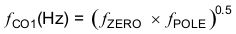Equation 46.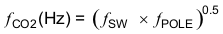Equation 47.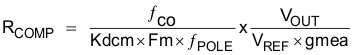Equation 48.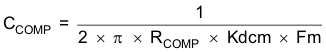Equation 49.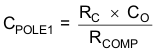Equation 50.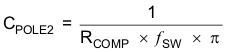#### 8.2.2.3 Application Curves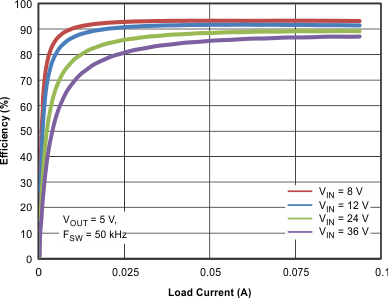Figure 39. Efficiency vs Load Current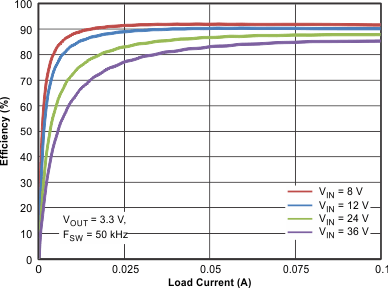Figure 41. Efficiency vs Load Current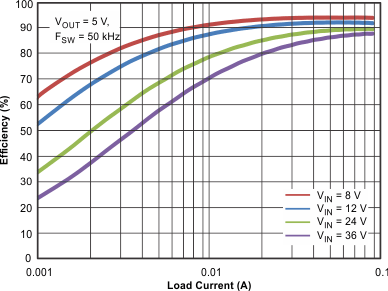Figure 40. Efficiency vs Load Current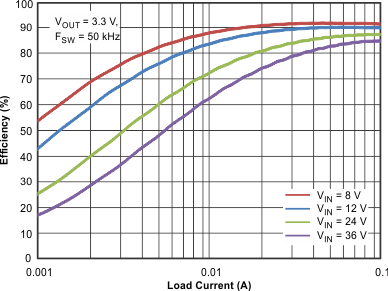Figure 42. Efficiency vs Load CurrentFigure 43. Frequency Response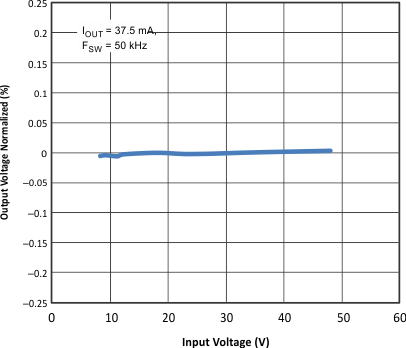Figure 45. Output Voltage Normalized vs Input VoltageFigure 47. Unload Transient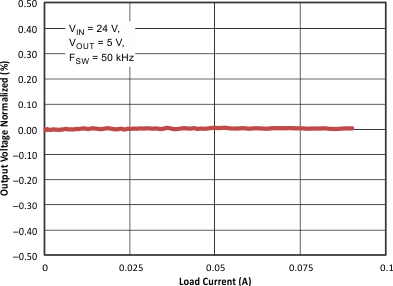Figure 44. Output Voltage Normalized vs Load Current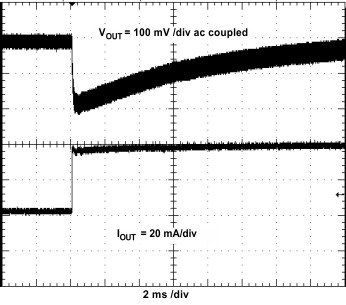Figure 46. Load Transient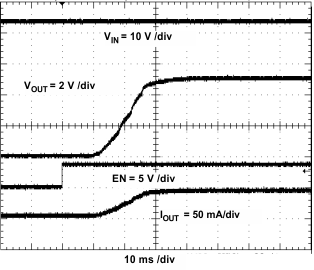Figure 48. Start-Up With ENA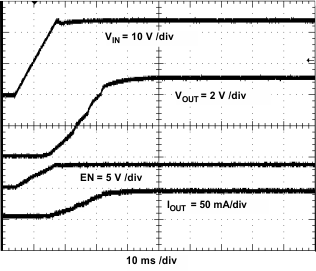Figure 49. Start-Up With VIN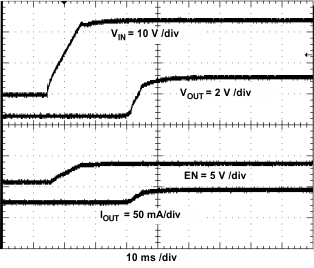Figure 51. Prebias Start-Up With VIN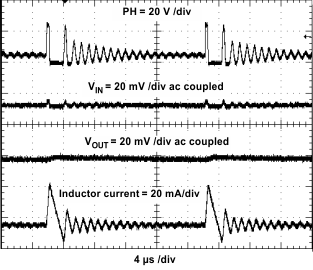Figure 53. Input and Output Ripple in PSMFigure 50. Prebias Start-Up With ENA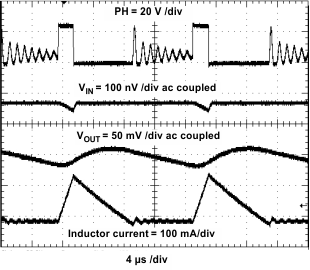Figure 52. Input and Output Ripple in DCM